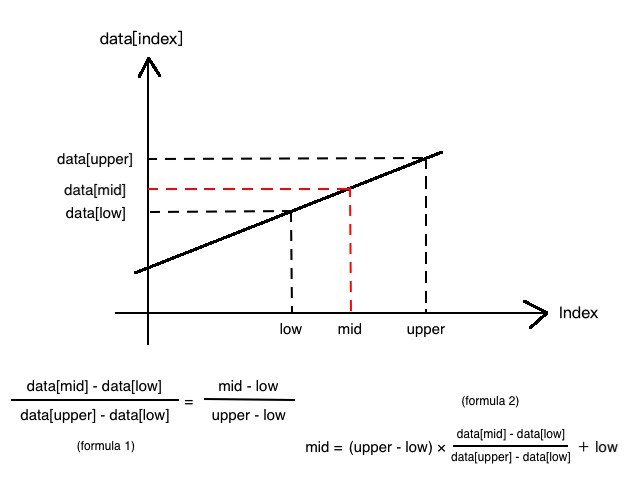#2019 iT 邦幫忙鐵人賽DAY 17
0
Software Development

## [演算法] 插補搜尋 (Interpolation Search)``````data = [1, 2, 3, 4, 5, 6, 7, 8, 9, 10]
def interpolation_search(data, key):
low = 0
upper = len(data) - 1
while low <= upper:
mid = int((upper - low) * (key - data[low]) / (data[upper] - data[low]) + low)
if mid < low or mid > upper:
break
if key < data[mid]:
upper = mid - 1
elif key > data[mid]:
low = mid + 1
else:
return mid

return -1

index = interpolation_search(data, 6)
if index >= 0:
print("找到數值於索引 " + str(index))
else:
print("找不到數值")
``````

30天學演算法和資料結構31